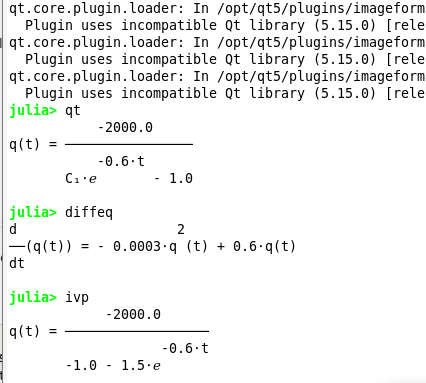# Sympy Computation with dsolve for Logistic Differential Equation

I was computing the solution for:
y' = ky(L-y)

the solution should be:

y(t) = C_{1} e^{0.6t} (2000 - y(t))

the value of C_{1} should be 2/3. Thus

y(t) = \frac{4000/3}{2/3 + e^{-0.6t}}

I believe I have followed the formula correctly and enter into the code, but it seems to be all wrong, this is the code:


using SymPy, Plots, LaTeXStrings, Plots.PlotMeasures
@syms q, t, r
@syms q()

# Computing IVP with SymPy
q(t).diff(t)
k = 0.0003
L = 2000
# Let Q' = kQ (L - Q)
diffeq = Eq(q(t).diff(t), k*q(t)*(L-q(t)) ); string(diffeq)
# To solve the ODE, pass it and the function to solve for to dsolve.
qt = dsolve(diffeq, q(t))
# To solve the Initial Value Problem q(0)=800
ivp = dsolve(diffeq, q(t), ics = Dict(q(0) => 800))

plot(y, 0,10,
legend=:topright, bottom_margin=5mm,
left_margin=5mm,
xlabel="years", ylabel="population",
label=L"y(t) = \frac{4000/3}{2/3 + e^{0.6 t}}",
size=(720, 360), tickfontsize=10)What did I do wrong or is it because Logistic Differential Equation can’t be solved with dsolve?

You might try it this way:

julia> @syms K, L, y(), t
(K, L, y, t)

julia> ∂ = Differential(t)
Differential(t)

julia> u = dsolve(∂(y(t)) ~ K*y(t)*(L - y(t)))
L⋅(C₁ + K⋅t)
L⋅ℯ
y(t) = ─────────────────
L⋅(C₁ + K⋅t)
ℯ             - 1

julia> ex =subs(rhs(u), L=>2000, K => 3//10000)
3⋅t
2000⋅C₁ + ───
5
2000⋅ℯ
───────────────────
3⋅t
2000⋅C₁ + ───
5
ℯ              - 1


If you have an initial condition for y(0) you can pass it to dsolve. If you want to substitute in for C_1 directly, you need to get that symbol. The command first(free_symbols(ex)) identifies it.

1 Like

I tried till this:


using SymPy, Plots, LaTeXStrings, Plots.PlotMeasures
@syms K, L, y(), t

∂ = Differential(t)
yt = dsolve(∂(y(t)) ~ K*y(t)*(L - y(t)))
sol_yt = subs(rhs(yt), L=>2000, K => 3//10000)

# Manual way
# Find the constant C₁
c1 = free_symbols(yt)
# To solve the Initial Value Problem, initial condition y(0) = 800
C1 = solve(sol_yt(t=>0) ~ 800, c1)
#ivp_yt = sol_yt(c1=>C1)


the computation to get constant C_{1} is taking too long.

But I made it with Python code, even if it is not Julia related here

# https://natelemoine.com/using-sympy-for-biological-odes/

import sympy as sm
import numpy as np
import matplotlib.pyplot as plt

from sympy.abc import L, k, t
y = sm.Function('y')(t)

# The differential equation: y' = ky(L-y)
# Define the derivative of y with respect to t (the left-hand side of the ODE),
# and then define the right-hand side of the ODE.
dy = y.diff(t)
rhs = k*y*(L - y)

# Define the equality
eq = sm.Eq(dy, rhs)
print('the Differential equation is:')
sm.pretty_print(eq)

print('the Differential equation solution is:')
sol = sm.dsolve(eq)
sm.pretty_print(sol)

print('the solution of the Differential equation for initial value of y(0) = 800:')

t0 = sol.args.subs({'t': 0})
n0 = 800
eq_init = sm.Eq(n0, t0)

C1 = t0.args.args.args
t0_sol = sm.solve(eq_init, C1)
# substitute the expression for C1 back into the equation
final = sol.args.subs(C1, t0_sol)
final_eq = final.simplify()
sm.pretty_print(final_eq.subs([(L,2000),(k,0.0003)]))

f_num = sm.lambdify([k, L, t], final_eq)
t_coords = np.linspace(0, 10, 100)
y_coords = f_num(0.0003, 2000, t_coords)

# Plot with SymPy Plotting Backends module:
sm.plot(final_eq.subs([(L,2000),(k,0.0003)]), (t, 0, 10),
xlabel='$t$ (year)', ylabel='$y(t)$ (population)', title='Logistic Differential Equation Plot')

# Plot with matplotlib
plt.figure()
plt.plot(t_coords, y_coords)
plt.title('Logistic Differential Equation Plot')
plt.xlabel("$t$ (year)")
plt.ylabel("$y(t)$ (population)")

plt.show()



If you know the initial value, you should let SymPy do the work within dsolve:

using SymPy
@syms K, L, y(), t
∂ = Differential(t)
yt = dsolve(∂(y(t)) ~ K*y(t)*(L - y(t)), ics=Dict(y(0) => 800)) # equation of t; K, L
yt = rhs(yt)    # work with just the expression, not the equation
yt = subs(yt, L=>2000, K => 3//10000)  # leaves as an expression of t alone


The value of yt can be passed on to plot using the recipe, or after calling lambdify can be passed as a function to other actions.

The ics argument allows you to pass a dictionary, as shown.

The earlier answer suggested what to do if you knew the value of C1 (find the variable, the substitute in for the known value). That isn’t suggested unless that is all you have to work with.

1 Like

I’m finally able to make it work with Julia code, thanks a lot

# https://discourse.julialang.org/t/sympy-computation-with-dsolve-for-logistic-differential-equation/104338/3

using SymPy, Plots, LaTeXStrings, Plots.PlotMeasures
@syms K, L, y(), t, y0

∂ = Differential(t)
yt_sym = dsolve(∂(y(t)) ~ K*y(t)*(L - y(t)), ics=Dict(y(0) => y0)) # equation of t; K, y0, L
yt_ivp = dsolve(∂(y(t)) ~ K*y(t)*(L - y(t)), ics=Dict(y(0) => 800)) # equation of t; K, L

yt = rhs(yt_ivp)    # work with just the expression, not the equation
yt_final = subs(yt, L=>2000, K => 3//10000)  # leaves as an expression of t alone

println("Logistic Differential Equation with Julia")
println("The solution")
sympy.pretty_print(yt_sym)

println("The solution for initial value problem y(0)=800")
sympy.pretty_print(yt_ivp)

println("Logistic Differential Equation with L=2000 and K = 0.00003")
sympy.pretty_print(yt_final)

plot(yt_final, 0,10,
legend=:bottomright, bottom_margin=5mm,
left_margin=5mm,
xlabel="years", ylabel="population",
label=L"y(t) = \frac{4000/3}{2/3 + e^{0.6 t}}",
size=(720, 360), tickfontsize=10)